# How diffusion models work: the math from scratch

Diffusion models are a new class of state-of-the-art generative models that generate diverse high-resolution images. They have already attracted a lot of attention after OpenAI, Nvidia and Google managed to train large-scale models. Example architectures that are based on diffusion models are GLIDE, DALLE-2, Imagen, and the full open-source stable diffusion.

But what is the main principle behind them?

In this blog post, we will dig our way up from the basic principles. There are already a bunch of different diffusion-based architectures. We will focus on the most prominent one, which is the Denoising Diffusion Probabilistic Models (DDPM) as initialized by Sohl-Dickstein et al and then proposed by Ho. et al 2020. Various other approaches will be discussed to a smaller extent such as stable diffusion and score-based models.

Diffusion models are fundamentally different from all the previous generative methods. Intuitively, they aim to decompose the image generation process (sampling) in many small “denoising” steps.

The intuition behind this is that the model can correct itself over these small steps and gradually produce a good sample. To some extent, this idea of refining the representation has already been used in models like alphafold. But hey, nothing comes at zero-cost. This iterative process makes them slow at sampling, at least compared to GANs.

## Diffusion process

The basic idea behind diffusion models is rather simple. They take the input image

$\mathbf{x}_0$

$T$

steps. We will call this the forward process. Notably, this is unrelated to the forward pass of a neural network. If you’d like, this part is necessary to generate the targets for our neural network (the image after applying

$t

Afterward, a neural network is trained to recover the original data by reversing the noising process. By being able to model the reverse process, we can generate new data. This is the so-called reverse diffusion process or, in general, the sampling process of a generative model.

How? Let’s dive into the math to make it crystal clear.

## Forward diffusion

Diffusion models can be seen as latent variable models. Latent means that we are referring to a hidden continuous feature space. In such a way, they may look similar to variational autoencoders (VAEs).

In practice, they are formulated using a Markov chain of

$T$

steps. Here, a Markov chain means that each step only depends on the previous one, which is a mild assumption. Importantly, we are not constrained to using a specific type of neural network, unlike flow-based models.

Given a data-point

$\textbf{x}_0$

$q(x)$

(

$\textbf{x}_0 \sim q(x)$

$\beta_{t}$

$\textbf{x}_{t-1}$

$\textbf{x}_{t}$

$q(\textbf{x}_t |\textbf{x}_{t-1})$

$q(\mathbf{x}_t \vert \mathbf{x}_{t-1}) = \mathcal{N}(\mathbf{x}_t; \boldsymbol{\mu}_t=\sqrt{1 – \beta_t} \mathbf{x}_{t-1}, \boldsymbol{\Sigma}_t = \beta_t\mathbf{I})$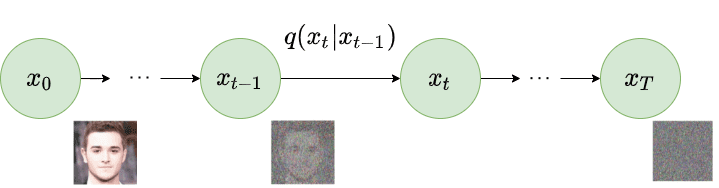Forward diffusion process. Image modified by Ho et al. 2020

Since we are in the multi-dimensional scenario

$\textbf{I}$

is the identity matrix, indicating that each dimension has the same standard deviation

$\beta_t$

$q(\mathbf{x}_t \vert \mathbf{x}_{t-1})$

$\boldsymbol{\mu}$

and the variance

$\boldsymbol{\Sigma}$

where

$\boldsymbol{\mu}_t =\sqrt{1 – \beta_t} \mathbf{x}_{t-1}$

$\boldsymbol{\Sigma}_t=\beta_t\mathbf{I}$

$\boldsymbol{\Sigma}$

will always be a diagonal matrix of variances (here

$\beta_t$

Thus, we can go in a closed form from the input data

$\mathbf{x}_0$

$\mathbf{x}_{T}$

$q(\mathbf{x}_{1:T} \vert \mathbf{x}_0) = \prod^T_{t=1} q(\mathbf{x}_t \vert \mathbf{x}_{t-1})$

The symbol

$:$

in

$q(\mathbf{x}_{1:T})$

$q$

repeatedly from timestep

$1$

to

$T$

. It’s also called trajectory.

So far, so good? Well, nah! For timestep

$t=500 < T$

$q$

500 times in order to sample

$\mathbf{x}_t$

The reparametrization trick provides a magic remedy to this.

### The reparameterization trick: tractable closed-form sampling at any timestep

If we define

$\alpha_t= 1- \beta_t$

$\bar{\alpha}_t = \prod_{s=0}^t \alpha_s$

$\boldsymbol{\epsilon}_{0},…, \epsilon_{t-2}, \epsilon_{t-1} \sim \mathcal{N}(\textbf{0},\mathbf{I})$

\begin{aligned}

\mathbf{x}_t

&=\sqrt{1 – \beta_t} \mathbf{x}_{t-1} + \sqrt{\beta_t}\boldsymbol{\epsilon}_{t-1}\\

&= \sqrt{\alpha_t}\mathbf{x}_{t-2} + \sqrt{1 – \alpha_t}\boldsymbol{\epsilon}_{t-2} \\

&= \dots \\

&= \sqrt{\bar{\alpha}_t}\mathbf{x}_0 + \sqrt{1 – \bar{\alpha}_t}\boldsymbol{\epsilon_0}

\end{aligned}

Note: Since all timestep have the same Gaussian noise we will only use the symbol

$\boldsymbol{\epsilon}$

from now on.

Thus to produce a sample

$\mathbf{x}_t$

$\mathbf{x}_t \sim q(\mathbf{x}_t \vert \mathbf{x}_0) = \mathcal{N}(\mathbf{x}_t; \sqrt{\bar{\alpha}_t} \mathbf{x}_0, (1 – \bar{\alpha}_t)\mathbf{I})$

Since

$\beta_t$

$\alpha_t$

$\bar{\alpha}_t$

$t$

and get

$\mathbf{x}_t$

$\mathbf{x}_t$

$L_t$

### Variance schedule

The variance parameter

$\beta_t$

$T$

timesteps. In fact, one can define a variance schedule, which can be linear, quadratic, cosine etc. The original DDPM authors utilized a linear schedule increasing from

$\beta_1= 10^{-4}$

$\beta_T = 0.02$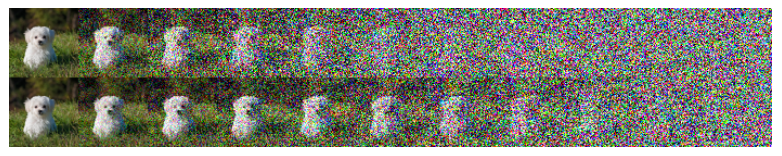Latent samples from linear (top) and cosine (bottom)
schedules respectively. Source: Nichol & Dhariwal 2021

## Reverse diffusion

As

$T \to \infty$

$x_T$

$q(\mathbf{x}_{t-1} \vert \mathbf{x}_{t})$

$x_T$

$\mathcal{N}(0,\mathbf{I})$

, run the reverse process and acquire a sample from

$q(x_0)$

The question is how we can model the reverse diffusion process.

### Approximating the reverse process with a neural network

In practical terms, we don’t know

$q(\mathbf{x}_{t-1} \vert \mathbf{x}_{t})$

$q(\mathbf{x}_{t-1} \vert \mathbf{x}_{t})$

$q(\mathbf{x}_{t-1} \vert \mathbf{x}_{t})$

$p_{\theta}$

$q(\mathbf{x}_{t-1} \vert \mathbf{x}_{t})$

$\beta_t$

$p_{\theta}$

$p_\theta(\mathbf{x}_{t-1} \vert \mathbf{x}_t) = \mathcal{N}(\mathbf{x}_{t-1}; \boldsymbol{\mu}_\theta(\mathbf{x}_t, t), \boldsymbol{\Sigma}_\theta(\mathbf{x}_t, t))$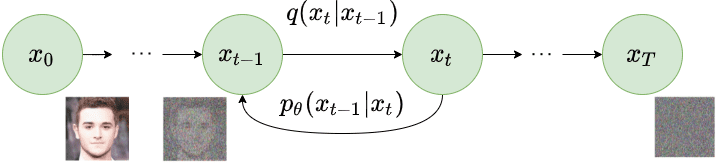Reverse diffusion process. Image modified by Ho et al. 2020

If we apply the reverse formula for all timesteps (

$p_\theta(\mathbf{x}_{0:T})$

$\mathbf{x}_T$

$p_\theta(\mathbf{x}_{0:T}) = p_{\theta}(\mathbf{x}_T) \prod^T_{t=1} p_\theta(\mathbf{x}_{t-1} \vert \mathbf{x}_t)$

By additionally conditioning the model on timestep

$t$

, it will learn to predict the Gaussian parameters (meaning the mean

$\boldsymbol{\mu}_\theta(\mathbf{x}_t, t)$

$\boldsymbol{\Sigma}_\theta(\mathbf{x}_t, t)$

But how do we train such a model?

## Training a diffusion model

If we take a step back, we can notice that the combination of

$q$

and

$p$

is very similar to a variational autoencoder (VAE). Thus, we can train it by optimizing the negative log-likelihood of the training data. After a series of calculations, which we won’t analyze here, we can write the evidence lower bound (ELBO) as follows:

\begin{aligned}

log p(\mathbf{x}) \geq

&\mathbb{E}_{q(x_1 \vert x_0)} [log p_{\theta} (\mathbf{x}_0 \vert \mathbf{x}_1)] – \\ &D_{KL}(q(\mathbf{x}_T \vert \mathbf{x}_0) \vert\vert p(\mathbf{x}_T))- \\

&\sum_{t=2}^T \mathbb{E}_{q(\mathbf{x}_t \vert \mathbf{x}_0)} [D_{KL}(q(\mathbf{x}_{t-1} \vert \mathbf{x}_t, \mathbf{x}_0) \vert \vert p_{\theta}(\mathbf{x}_{t-1} \vert \mathbf{x}_t)) ] \\

& = L_0 – L_T – \sum_{t=2}^T L_{t-1}

\end{aligned}

Let’s analyze these terms:

1. The

$\mathbb{E}_{q(x_1 \vert x_0)} [log p_{\theta} (\mathbf{x}_0 \vert \mathbf{x}_1)]$

2. $D_{KL}(q(\mathbf{x}_T \vert \mathbf{x}_0) \vert\vert p(\mathbf{x}_T))$

$\mathbf{x}_T$

3. The third term

$\sum_{t=2}^T L_{t-1}$

$L_t$

$p_{\theta}(\mathbf{x}_{t-1} \vert \mathbf{x}_t))$

$q(\mathbf{x}_{t-1} \vert \mathbf{x}_t, \mathbf{x}_0)$

It is evident that through the ELBO, maximizing the likelihood boils down to learning the denoising steps

$L_t$

Important note: Even though

$q(\mathbf{x}_{t-1} \vert \mathbf{x}_{t})$

$\textbf{x}_0$

Intuitively, a painter (our generative model) needs a reference image (

$\textbf{x}_0$

$q(\mathbf{x}_{t-1} \vert \mathbf{x}_t, \mathbf{x}_0)$

$\textbf{x}_0$

In other words, we can sample

$\textbf{x}_t$

$t$

conditioned on

$\textbf{x}_0$

$\alpha_t= 1- \beta_t$

$\bar{\alpha}_t = \prod_{s=0}^t \alpha_s$

\begin{aligned}

q(\mathbf{x}_{t-1} \vert \mathbf{x}_t, \mathbf{x}_0) &= \mathcal{N}(\mathbf{x}_{t-1}; {\tilde{\boldsymbol{\mu}}}(\mathbf{x}_t, \mathbf{x}_0), {\tilde{\beta}_t} \mathbf{I}) \\

\tilde{\beta}_t &= \frac{1 – \bar{\alpha}_{t-1}}{1 – \bar{\alpha}_t} \cdot \beta_t \\

\tilde{\boldsymbol{\mu}}_t (\mathbf{x}_t, \mathbf{x}_0) &= \frac{\sqrt{\bar{\alpha}_{t-1}}\beta_t}{1 – \bar{\alpha}_t} \mathbf{x_0} + \frac{\sqrt{\alpha_t}(1 – \bar{\alpha}_{t-1})}{1 – \bar{\alpha}_t} \mathbf{x}_t

\end{aligned}

Note that

$\alpha_t$

$\bar{\alpha}_t$

$\beta_t$

This little trick provides us with a fully tractable ELBO. The above property has one more important side effect, as we already saw in the reparameterization trick, we can represent

$\mathbf{x}_0$

$\mathbf{x}_0 = \frac{1}{\sqrt{\bar{\alpha}_t}}(\mathbf{x}_t – \sqrt{1 – \bar{\alpha}_t} \boldsymbol{\epsilon})),$

where

$\boldsymbol{\epsilon} \sim \mathcal{N}(\textbf{0},\mathbf{I})$

By combining the last two equations, each timestep will now have a mean

$\tilde{\boldsymbol{\mu}}_t$

$\mathbf{x}_t$

$\tilde{\boldsymbol{\mu}}_t (\mathbf{x}_t) = {\frac{1}{\sqrt{\alpha_t}} \Big( \mathbf{x}_t – \frac{\beta_t}{\sqrt{1 – \bar{\alpha}_t}} \boldsymbol{\epsilon} ) \Big)}$

Therefore we can use a neural network

$\epsilon_{\theta}(\mathbf{x}_t,t)$

$\boldsymbol{\epsilon}$

and consequently the mean:

$\tilde{\boldsymbol{\mu}_{\theta}}( \mathbf{x}_t,t) = {\frac{1}{\sqrt{\alpha_t}} \Big( \mathbf{x}_t – \frac{\beta_t}{\sqrt{1 – \bar{\alpha}_t}} \boldsymbol{\epsilon}_{\theta}(\mathbf{x}_t,t) \Big)}$

Thus, the loss function (the denoising term in the ELBO) can be expressed as:

\begin{aligned}

L_t &= \mathbb{E}_{\mathbf{x}_0,t,\boldsymbol{\epsilon}}\Big[\frac{1}{2||\boldsymbol{\Sigma}_\theta (x_t,t)||_2^2} ||\tilde{\boldsymbol{\mu}}_t – \boldsymbol{\mu}_\theta(\mathbf{x}_t, t)||_2^2 \Big] \\

&= \mathbb{E}_{\mathbf{x}_0,t,\boldsymbol{\epsilon}}\Big[\frac{\beta_t^2}{2\alpha_t (1 – \bar{\alpha}_t) ||\boldsymbol{\Sigma}_\theta||^2_2} \| \boldsymbol{\epsilon}_{t}- \boldsymbol{\epsilon}_{\theta}(\sqrt{\bar{a}_t} \mathbf{x}_0 + \sqrt{1-\bar{a}_t}\boldsymbol{\epsilon}, t ) ||^2 \Big]

\end{aligned}

This effectively shows us that instead of predicting the mean of the distribution, the model will predict the noise

$\boldsymbol{\epsilon}$

at each timestep

$t$

.

Ho et.al 2020 made a few simplifications to the actual loss term as they ignore a weighting term. The simplified version outperforms the full objective:

$L_t^\text{simple} = \mathbb{E}_{\mathbf{x}_0, t, \boldsymbol{\epsilon}} \Big[\|\boldsymbol{\epsilon}- \boldsymbol{\epsilon}_{\theta}(\sqrt{\bar{a}_t} \mathbf{x}_0 + \sqrt{1-\bar{a}_t} \boldsymbol{\epsilon}, t ) ||^2 \Big]$

The authors found that optimizing the above objective works better than optimizing the original ELBO. The proof for both equations can be found in this excellent post by Lillian Weng or in Luo et al. 2022.

Additionally, Ho et. al 2020 decide to keep the variance fixed and have the network learn only the mean. This was later improved by Nichol et al. 2021, who decide to let the network learn the covariance matrix

$(\boldsymbol{\Sigma})$

as well (by modifying

$L_t^\text{simple}$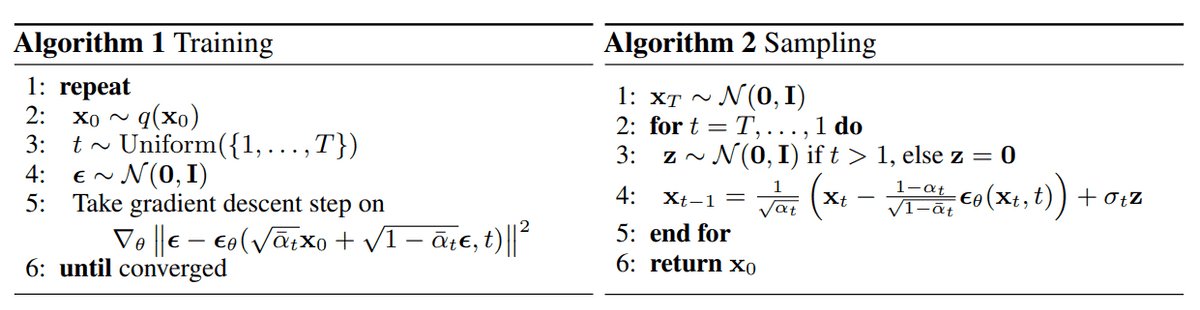Training and sampling algorithms of DDPMs. Source: Ho et al. 2020

## Architecture

One thing that we haven’t mentioned so far is what the model’s architecture looks like. Notice that the model’s input and output should be of the same size.

To this end, Ho et al. employed a U-Net. If you are unfamiliar with U-Nets, feel free to check out our past article on the major U-Net architectures. In a few words, a U-Net is a symmetric architecture with input and output of the same spatial size that uses skip connections between encoder and decoder blocks of corresponding feature dimension. Usually, the input image is first downsampled and then upsampled until reaching its initial size.

In the original implementation of DDPMs, the U-Net consists of Wide ResNet blocks, group normalization as well as self-attention blocks.

The diffusion timestep

$t$

is specified by adding a sinusoidal position embedding into each residual block. For more details, feel free to visit the official GitHub repository. For a detailed implementation of the diffusion model, check out this awesome post by Hugging Face.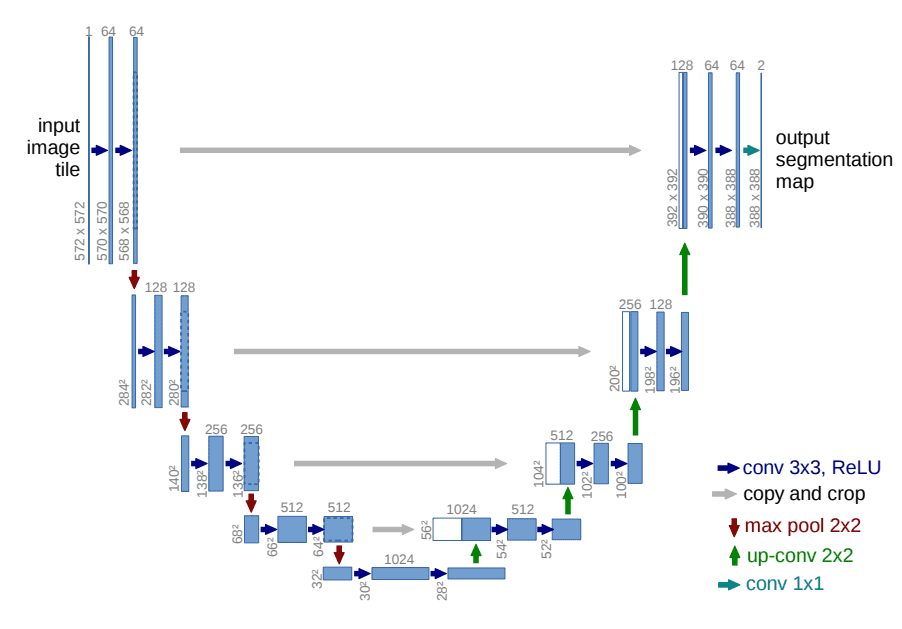The U-Net architecture. Source: Ronneberger et al.

## Conditional Image Generation: Guided Diffusion

A crucial aspect of image generation is conditioning the sampling process to manipulate the generated samples. Here, this is also referred to as guided diffusion.

There have even been methods that incorporate image embeddings into the diffusion in order to “guide” the generation. Mathematically, guidance refers to conditioning a prior data distribution

$p(\textbf{x})$

with a condition

$y$

, i.e. the class label or an image/text embedding, resulting in

$p(\textbf{x}|y)$

.

To turn a diffusion model

$p_\theta$

$y$

at each diffusion step.

$p_\theta(\mathbf{x}_{0:T} \vert y) = p_\theta(\mathbf{x}_T) \prod^T_{t=1} p_\theta(\mathbf{x}_{t-1} \vert \mathbf{x}_t, y)$

The fact that the conditioning is being seen at each timestep may be a good justification for the excellent samples from a text prompt.

In general, guided diffusion models aim to learn

$\nabla \log p_\theta( \mathbf{x}_t \vert y)$

\begin{aligned}

\nabla_{\textbf{x}_{t}} \log p_\theta(\mathbf{x}_t \vert y) &= \nabla_{\textbf{x}_{t}} \log (\frac{p_\theta(y \vert \mathbf{x}_t) p_\theta(\mathbf{x}_t) }{p_\theta(y)}) \\

&= \nabla_{\textbf{x}_{t}} log p_\theta(\mathbf{x}_t) + \nabla_{\textbf{x}_{t}} log (p_\theta( y \vert\mathbf{x}_t ))

\end{aligned}

$p_\theta(y)$

$\nabla_{\textbf{x}_{t}}$

$\textbf{x}_{t}$

$y$

. Moreover remember that

$\log(a b)= \log(a) + \log(b)$

And by adding a guidance scalar term

$s$

, we have:

$\nabla \log p_\theta(\mathbf{x}_t \vert y) = \nabla \log p_\theta(\mathbf{x}_t) + s \cdot \nabla \log (p_\theta( y \vert\mathbf{x}_t ))$

Using this formulation, let’s make a distinction between classifier and classifier-free guidance. Next, we will present two family of methods aiming at injecting label information.

### Classifier guidance

Sohl-Dickstein et al. and later Dhariwal and Nichol showed that we can use a second model, a classifier

$f_\phi(y \vert \mathbf{x}_t, t)$

$y$

during training. To achieve that, we can train a classifier

$f_\phi(y \vert \mathbf{x}_t, t)$

$\mathbf{x}_t$

$y$

. Then we can use the gradients

$\nabla \log (f_\phi( y \vert\mathbf{x}_t ))$

We can build a class-conditional diffusion model with mean

$\mu_\theta(\mathbf{x}_t|y)$

$\boldsymbol{\Sigma}_\theta(\mathbf{x}_t |y)$

Since

$p_\theta \sim \mathcal{N}(\mu_{\theta}, \Sigma_{\theta})$

$\log f_\phi(y|\mathbf{x}_t)$

$y$

, resulting in:

$\hat{\mu}(\mathbf{x}_t |y) =\mu_\theta(\mathbf{x}_t |y) + s \cdot \boldsymbol{\Sigma}_\theta(\mathbf{x}_t |y) \nabla_{\mathbf{x}_t} logf_\phi(y \vert \mathbf{x}_t, t)$

In the famous GLIDE paper by Nichol et al, the authors expanded on this idea and use CLIP embeddings to guide the diffusion. CLIP as proposed by Saharia et al., consists of an image encoder

$g$

and a text encoder

$h$

. It produces an image and text embeddings

$g(\mathbf{x}_t)$

$h(c)$

, respectively, wherein

$c$

is the text caption.

Therefore, we can perturb the gradients with their dot product:

$\hat{\mu}(\mathbf{x}_t |c) =\mu(\mathbf{x}_t |c) + s \cdot \boldsymbol{\Sigma}_\theta(\mathbf{x}_t |c) \nabla_{\mathbf{x}_t} g(\mathbf{x}_t) \cdot h(c)$

As a result, they manage to “steer” the generation process toward a user-defined text caption.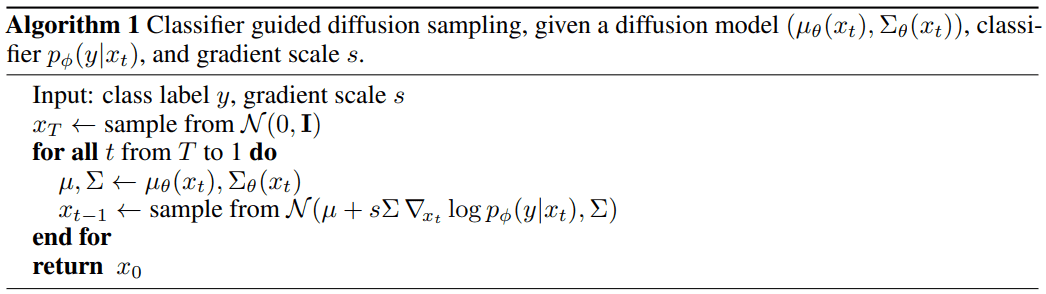Algorithm of classifier guided diffusion sampling. Source: Dhariwal & Nichol 2021

### Classifier-free guidance

Using the same formulation as before we can define a classifier-free guided diffusion model as:

$\nabla \log p(\mathbf{x}_t \vert y) =s \cdot \nabla log(p(\mathbf{x}_t \vert y)) + (1-s) \cdot \nabla log p(\mathbf{x}_t)$

Guidance can be achieved without a second classifier model as proposed by Ho & Salimans. Instead of training a separate classifier, the authors trained a conditional diffusion model

$\boldsymbol{\epsilon}_\theta (\mathbf{x}_t|y)$

$\boldsymbol{\epsilon}_\theta (\mathbf{x}_t |0)$

$y$

to

$0$

, so that the model is exposed to both the conditional and unconditional setup:

\begin{aligned}

\hat{\boldsymbol{\epsilon}}_\theta(\mathbf{x}_t |y) & = s \cdot \boldsymbol{\epsilon}_\theta(\mathbf{x}_t |y) + (1-s) \cdot \boldsymbol{\epsilon}_\theta(\mathbf{x}_t |0) \\

&= \boldsymbol{\epsilon}_\theta(\mathbf{x}_t |0) + s \cdot (\boldsymbol{\epsilon}_\theta(\mathbf{x}_t |y) -\boldsymbol{\epsilon}_\theta(\mathbf{x}_t |0) )

\end{aligned}

Note that this can also be used to “inject” text embeddings as we showed in classifier guidance.

• It uses only a single model to guide the diffusion.

• It simplifies guidance when conditioning on information that is difficult to predict with a classifier (such as text embeddings).

Imagen as proposed by Saharia et al. relies heavily on classifier-free guidance, as they find that it is a key contributor to generating samples with strong image-text alignment. For more info on the approach of Imagen check out this video from AI Coffee Break with Letitia:

## Scaling up diffusion models

You might be asking what is the problem with these models. Well, it’s computationally very expensive to scale these U-nets into high-resolution images. This brings us to two methods for scaling up diffusion models to higher resolutions: cascade diffusion models and latent diffusion models.

Ho et al. 2021 introduced cascade diffusion models in an effort to produce high-fidelity images. A cascade diffusion model consists of a pipeline of many sequential diffusion models that generate images of increasing resolution. Each model generates a sample with superior quality than the previous one by successively upsampling the image and adding higher resolution details. To generate an image, we sample sequentially from each diffusion model.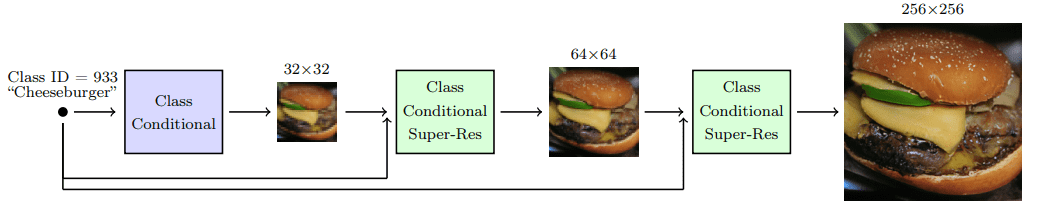Cascade diffusion model pipeline. Source: Ho & Saharia et al.

To acquire good results with cascaded architectures, strong data augmentations on the input of each super-resolution model are crucial. Why? Because it alleviates compounding error from the previous cascaded models, as well as due to a train-test mismatch.

It was found that gaussian blurring is a critical transformation toward achieving high fidelity. They refer to this technique as conditioning augmentation.

### Stable diffusion: Latent diffusion models

Latent diffusion models are based on a rather simple idea: instead of applying the diffusion process directly on a high-dimensional input, we project the input into a smaller latent space and apply the diffusion there.

In more detail, Rombach et al. proposed to use an encoder network to encode the input into a latent representation i.e.

$\mathbf{z}_t = g(\mathbf{x}_t)$

If the loss for a typical diffusion model (DM) is formulated as:

$L _{DM} = \mathbb{E}_{\mathbf{x}, t, \boldsymbol{\epsilon}} \Big[\| \boldsymbol{\epsilon}- \boldsymbol{\epsilon}_{\theta}( \mathbf{x}_t, t ) ||^2 \Big]$

then given an encoder

$\mathcal{E}$

and a latent representation

$z$

, the loss for a latent diffusion model (LDM) is:

$L _{LDM} = \mathbb{E}_{ \mathcal{E}(\mathbf{x}), t, \boldsymbol{\epsilon}} \Big[\| \boldsymbol{\epsilon}- \boldsymbol{\epsilon}_{\theta}( \mathbf{z}_t, t ) ||^2 \Big]$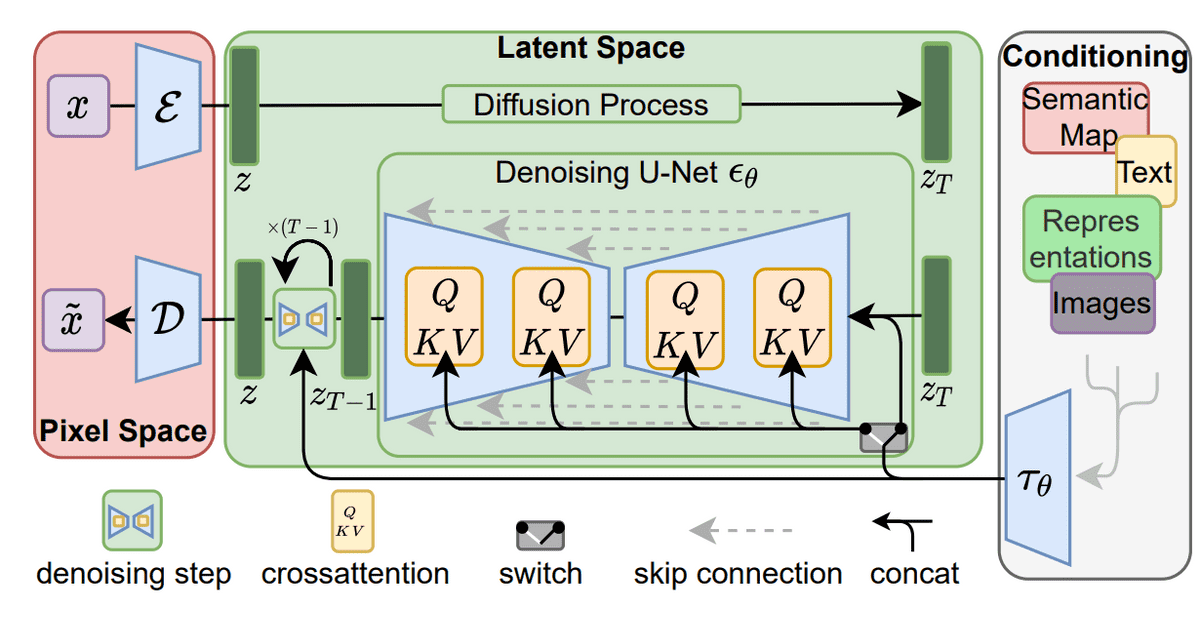Latent diffusion models. Source: Rombach et al

## Score-based generative models

Around the same time as the DDPM paper, Song and Ermon proposed a different type of generative model that appears to have many similarities with diffusion models. Score-based models tackle generative learning using score matching and Langevin dynamics.

Score-matching refers to the process of modeling the gradient of the log probability density function, also known as the score function. Langevin dynamics is an iterative process that can draw samples from a distribution using only its score function.

$\mathbf{x}_t=\mathbf{x}_{t-1}+\frac{\delta}{2} \nabla_{\mathbf{x}} \log p\left(\mathbf{x}_{t-1}\right)+\sqrt{\delta} \boldsymbol{\epsilon}, \quad \text { where } \boldsymbol{\epsilon} \sim \mathcal{N}(\mathbf{0}, \mathbf{I})$

where

$\delta$

is the step size.

Suppose that we have a probability density

$p(x)$

and that we define the score function to be

$\nabla_x \log p(x)$

$s_{\theta}$

$\nabla_x \log p(x)$

$p(x)$

first. The training objective can be formulated as follows:

$\mathbb{E}_{p(\mathbf{x})}[\| \nabla_\mathbf{x} \log p(\mathbf{x}) – \mathbf{s}_\theta(\mathbf{x}) \|_2^2] = \int p(\mathbf{x}) \| \nabla_\mathbf{x} \log p(\mathbf{x}) – \mathbf{s}_\theta(\mathbf{x}) \|_2^2 \mathrm{d}\mathbf{x}$

Then by using Langevin dynamics, we can directly sample from

$p(x)$

using the approximated score function.

In case you missed it, guided diffusion models use this formulation of score-based models as they learn directly

$\nabla_x \log p(x)$

### Adding noise to score-based models: Noise Conditional Score Networks (NCSN)

The problem so far: the estimated score functions are usually inaccurate in low-density regions, where few data points are available. As a result, the quality of data sampled using Langevin dynamics is not good.

Their solution was to perturb the data points with noise and train score-based models on the noisy data points instead. As a matter of fact, they used multiple scales of Gaussian noise perturbations.

Thus, adding noise is the key to make both DDPM and score based models work.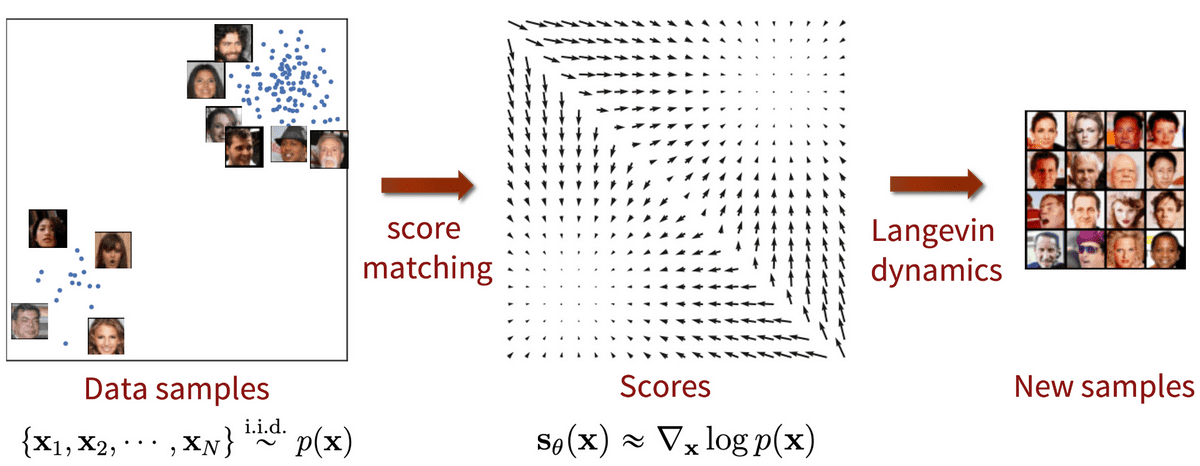Score-based generative modeling with score matching + Langevin dynamics. Source: Generative Modeling by Estimating Gradients of the Data Distribution

Mathematically, given the data distribution

$p(x)$

, we perturb with Gaussian noise

$\mathcal{N}(\textbf{0}, \sigma_i^2 I)$

$i=1,2,\cdots,L$

$p_{\sigma_i}(\mathbf{x}) = \int p(\mathbf{y}) \mathcal{N}(\mathbf{x}; \mathbf{y}, \sigma_i^2 I) \mathrm{d} \mathbf{y}$

Then we train a network

$s_\theta(\mathbf{x},i)$

$\nabla_\mathbf{x} \log d_{\sigma_i}(\mathbf{x})$

$\sum_{i=1}^L \lambda(i) \mathbb{E}_{p_{\sigma_i}(\mathbf{x})}[\| \nabla_\mathbf{x} \log p_{\sigma_i}(\mathbf{x}) – \mathbf{s}_\theta(\mathbf{x}, i) \|_2^2]$

### Score-based generative modeling through stochastic differential equations (SDE)

Song et al. 2021 explored the connection of score-based models with diffusion models. In an effort to encapsulate both NSCNs and DDPMs under the same umbrella, they proposed the following:

Instead of perturbing data with a finite number of noise distributions, we use a continuum of distributions that evolve over time according to a diffusion process. This process is modeled by a prescribed stochastic differential equation (SDE) that does not depend on the data and has no trainable parameters. By reversing the process, we can generate new samples.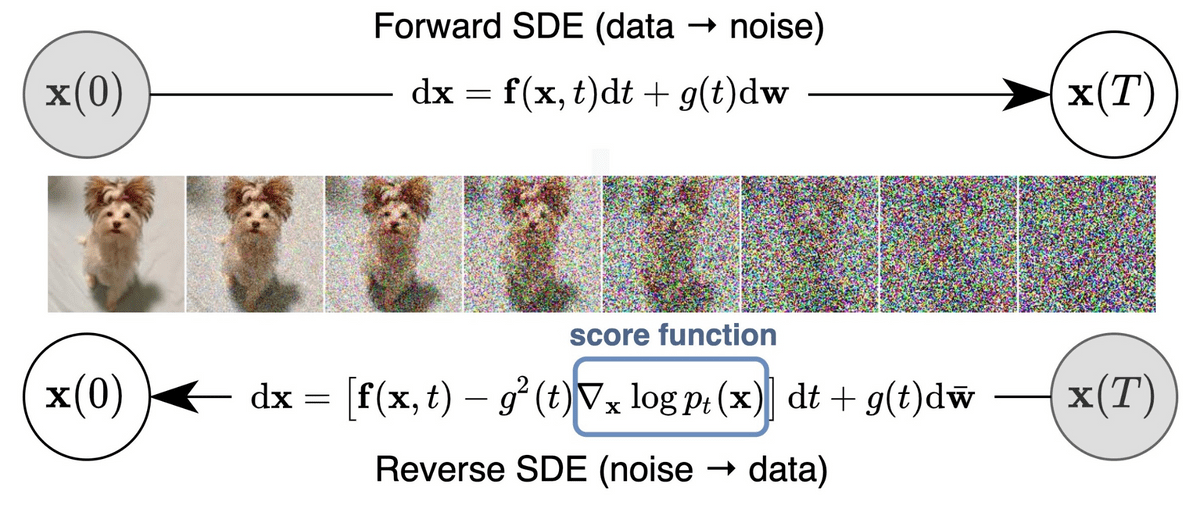Score-based generative modeling through stochastic differential equations (SDE). Source: Song et al. 2021

We can define the diffusion process

$\{ \mathbf{x}$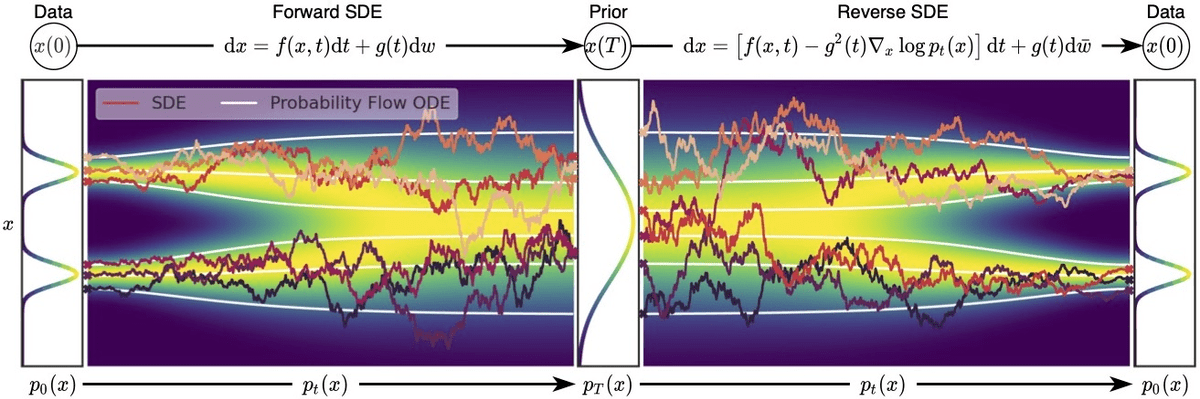Overview of score-based generative modeling through SDEs. Source: Song et al. 2021

## Summary

Let’s do a quick sum-up of the main points we learned in this blogpost:

• Diffusion models work by gradually adding gaussian noise through a series of

$T$

steps into the original image, a process known as diffusion.

• To sample new data, we approximate the reverse diffusion process using a neural network.

• The training of the model is based on maximizing the evidence lower bound (ELBO).

• We can condition the diffusion models on image labels or text embeddings in order to “guide” the diffusion process.

• Cascade and Latent diffusion are two approaches to scale up models to high-resolutions.

• Cascade diffusion models are sequential diffusion models that generate images of increasing resolution.

• Latent diffusion models (like stable diffusion) apply the diffusion process on a smaller latent space for computational efficiency using a variational autoencoder for the up and downsampling.

• Score-based models also apply a sequence of noise perturbations to the original image. But they are trained using score-matching and Langevin dynamics. Nonetheless, they end up in a similar objective.

• The diffusion process can be formulated as an SDE. Solving the reverse SDE allows us to generate new samples.

Finally, for more associations between diffusion models and VAE or AE check out these really nice blogs.

## Cite as

@article{karagiannakos2022diffusionmodels,    title   = "Diffusion models: toward state-of-the-art image generation",    author  = "Karagiannakos, Sergios, Adaloglou, Nikolaos",    journal = "https://theaisummer.com/",    year    = "2022",    howpublished = {https://theaisummer.com/diffusion-models/},  }

 Sohl-Dickstein, Jascha, et al. Deep Unsupervised Learning Using Nonequilibrium Thermodynamics. arXiv:1503.03585, arXiv, 18 Nov. 2015

 Ho, Jonathan, et al. Denoising Diffusion Probabilistic Models. arXiv:2006.11239, arXiv, 16 Dec. 2020

 Nichol, Alex, and Prafulla Dhariwal. Improved Denoising Diffusion Probabilistic Models. arXiv:2102.09672, arXiv, 18 Feb. 2021

 Dhariwal, Prafulla, and Alex Nichol. Diffusion Models Beat GANs on Image Synthesis. arXiv:2105.05233, arXiv, 1 June 2021

 Nichol, Alex, et al. GLIDE: Towards Photorealistic Image Generation and Editing with Text-Guided Diffusion Models. arXiv:2112.10741, arXiv, 8 Mar. 2022

 Ho, Jonathan, and Tim Salimans. Classifier-Free Diffusion Guidance. 2021. openreview.net

 Ramesh, Aditya, et al. Hierarchical Text-Conditional Image Generation with CLIP Latents. arXiv:2204.06125, arXiv, 12 Apr. 2022

 Saharia, Chitwan, et al. Photorealistic Text-to-Image Diffusion Models with Deep Language Understanding. arXiv:2205.11487, arXiv, 23 May 2022

 Rombach, Robin, et al. High-Resolution Image Synthesis with Latent Diffusion Models. arXiv:2112.10752, arXiv, 13 Apr. 2022

 Ho, Jonathan, et al. Cascaded Diffusion Models for High Fidelity Image Generation. arXiv:2106.15282, arXiv, 17 Dec. 2021

 Weng, Lilian. What Are Diffusion Models? 11 July 2021

 O’Connor, Ryan. Introduction to Diffusion Models for Machine Learning AssemblyAI Blog, 12 May 2022

 Rogge, Niels and Rasul, Kashif. The Annotated Diffusion Model . Hugging Face Blog, 7 June 2022

 Das, Ayan. “An Introduction to Diffusion Probabilistic Models.” Ayan Das, 4 Dec. 2021

 Song, Yang, and Stefano Ermon. Generative Modeling by Estimating Gradients of the Data Distribution. arXiv:1907.05600, arXiv, 10 Oct. 2020

 Song, Yang, and Stefano Ermon. Improved Techniques for Training Score-Based Generative Models. arXiv:2006.09011, arXiv, 23 Oct. 2020

 Song, Yang, et al. Score-Based Generative Modeling through Stochastic Differential Equations. arXiv:2011.13456, arXiv, 10 Feb. 2021

 Song, Yang. Generative Modeling by Estimating Gradients of the Data Distribution, 5 May 2021

 Luo, Calvin. Understanding Diffusion Models: A Unified Perspective. 25 Aug. 2022